Ex 3.1

Chapter 3 Class 6 Playing with Numbers
Serial order wise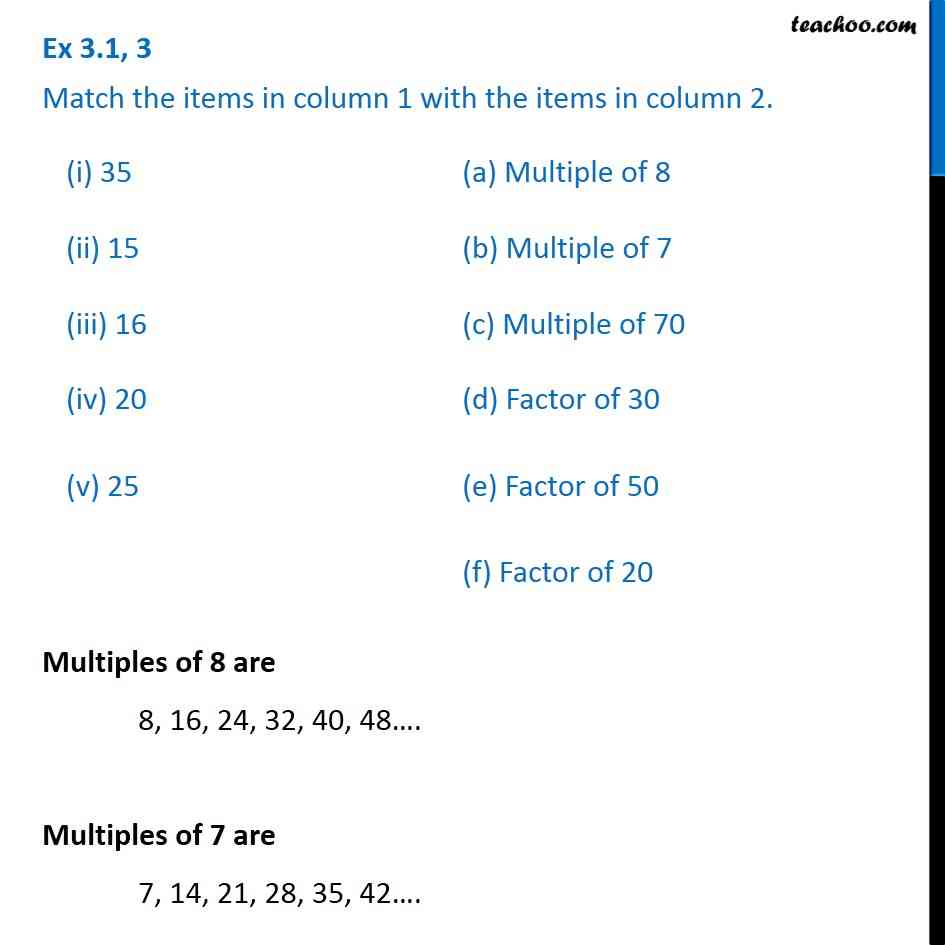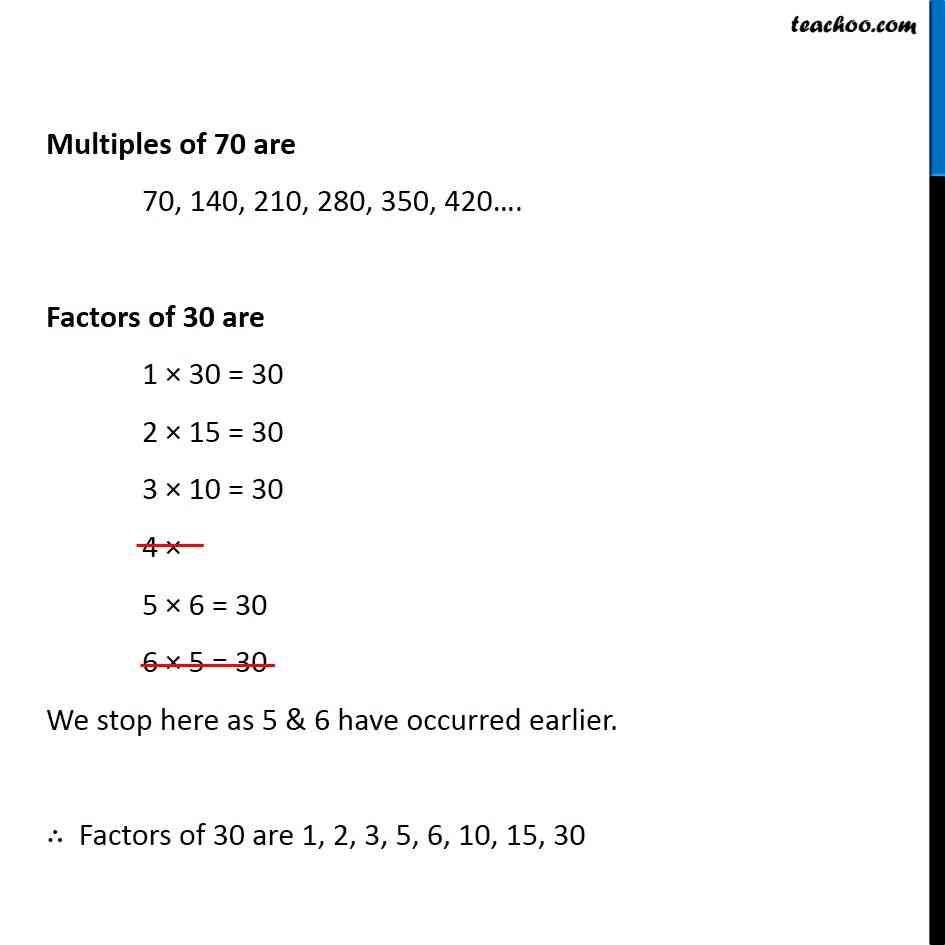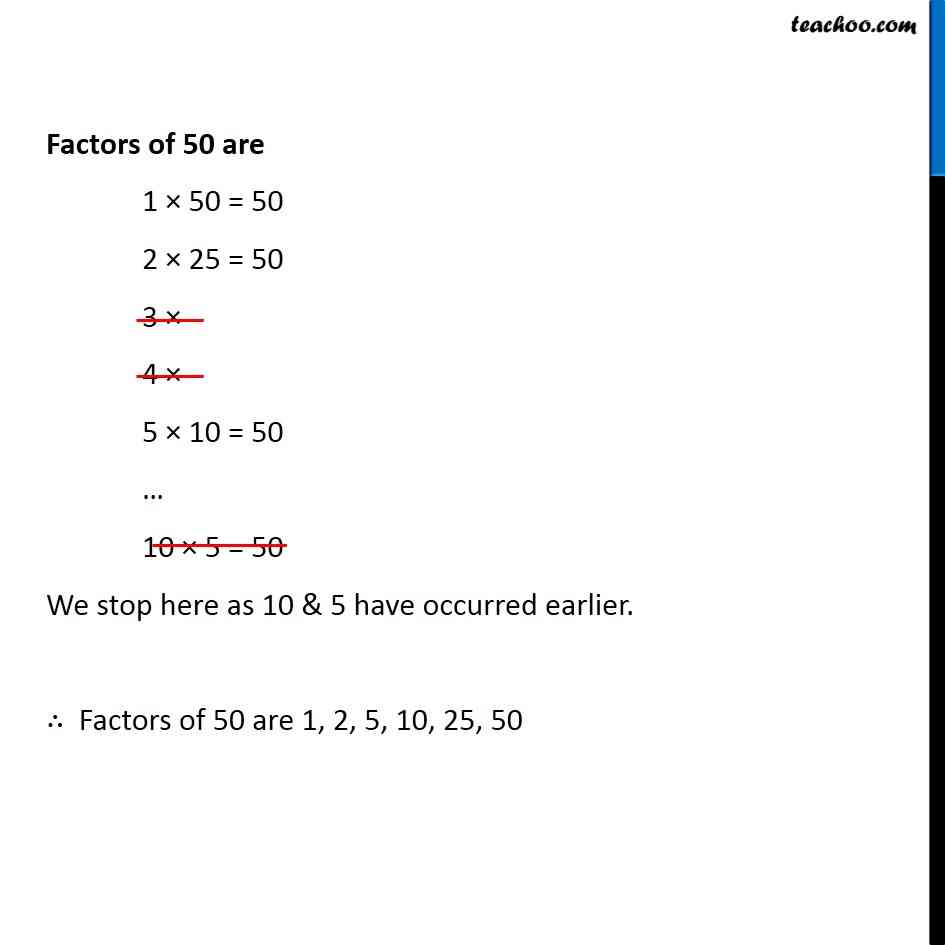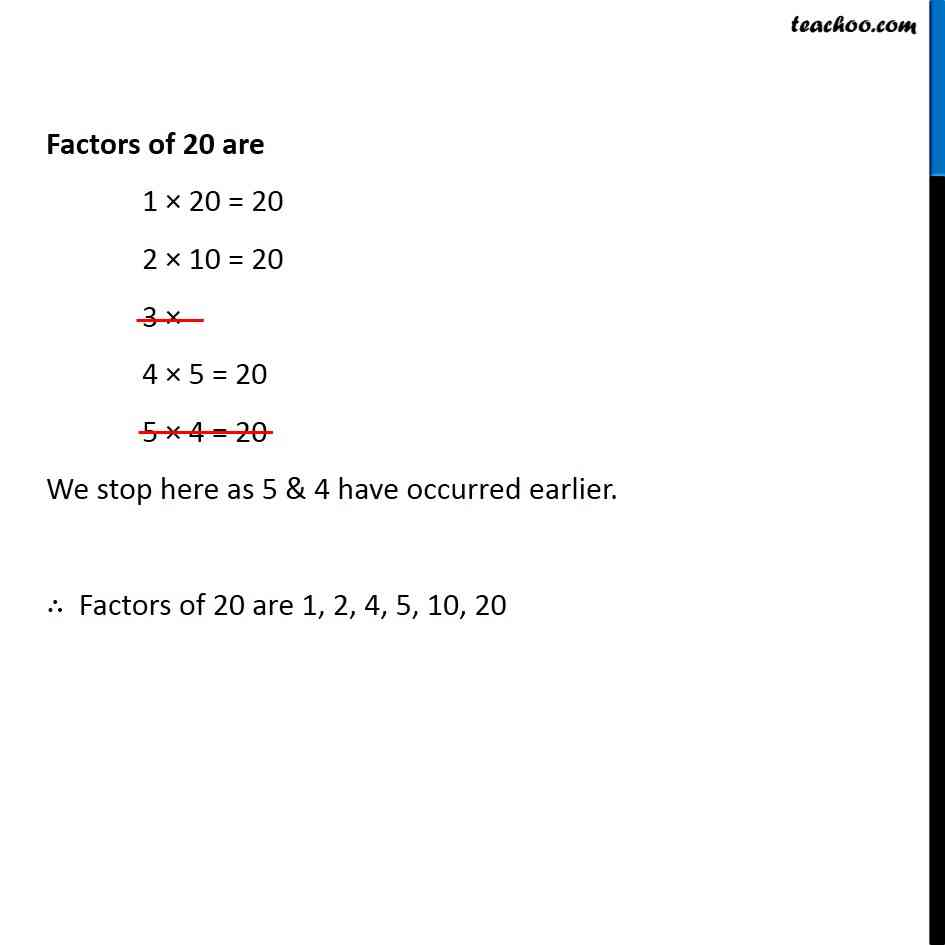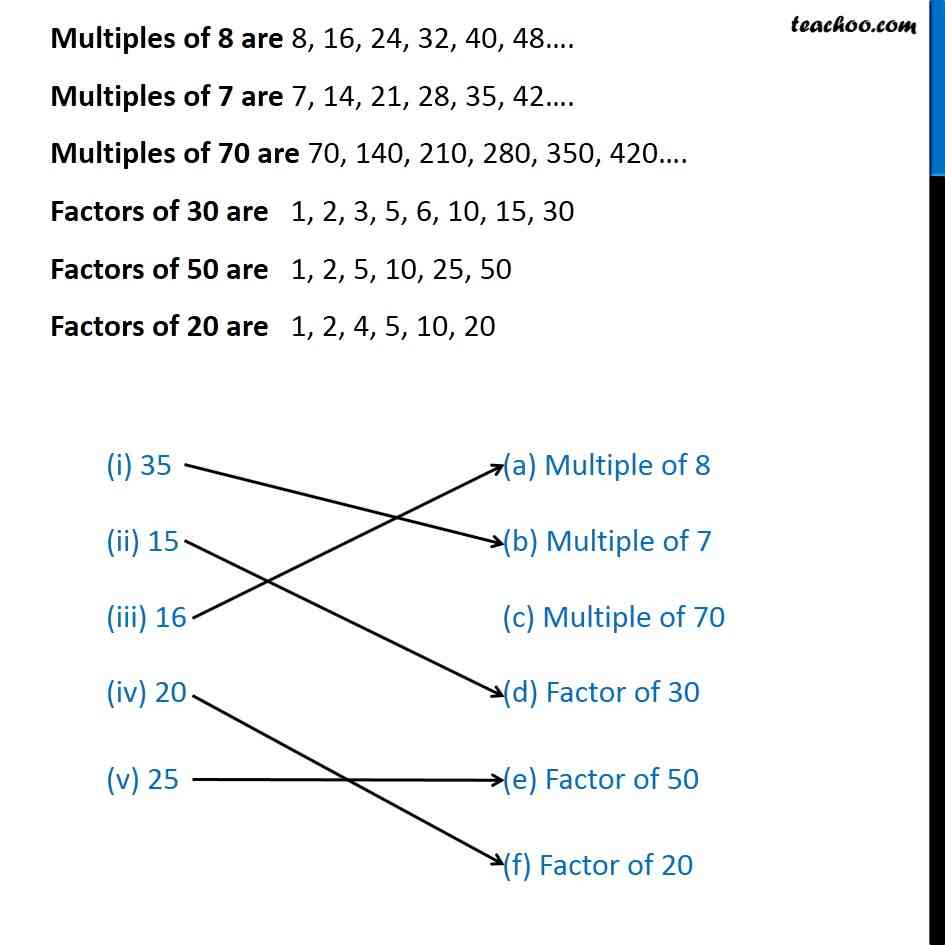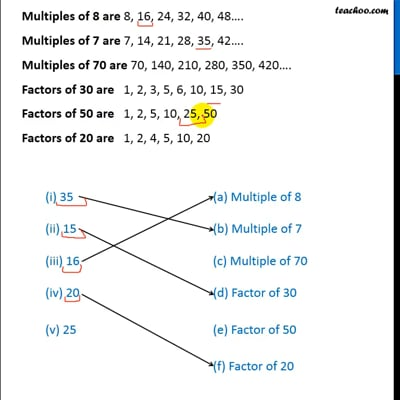This video is only available for Teachoo black users

### Transcript

Ex 3.1, 3 Match the items in column 1 with the items in column 2. Multiples of 8 are 8, 16, 24, 32, 40, 48…. Multiples of 7 are 7, 14, 21, 28, 35, 42…. Multiples of 70 are 70, 140, 210, 280, 350, 420…. Factors of 30 are 1 × 30 = 30 2 × 15 = 30 3 × 10 = 30 4 × 5 × 6 = 30 6 × 5 = 30 We stop here as 5 & 6 have occurred earlier. ∴ Factors of 30 are 1, 2, 3, 5, 6, 10, 15, 30 Factors of 50 are 1 × 50 = 50 2 × 25 = 50 3 × 4 × 5 × 10 = 50 … 10 × 5 = 50 We stop here as 10 & 5 have occurred earlier. ∴ Factors of 50 are 1, 2, 5, 10, 25, 50 Factors of 20 are 1 × 20 = 20 2 × 10 = 20 3 × 4 × 5 = 20 5 × 4 = 20 We stop here as 5 & 4 have occurred earlier. ∴ Factors of 20 are 1, 2, 4, 5, 10, 20 Multiples of 8 are 8, 16, 24, 32, 40, 48…. Multiples of 7 are 7, 14, 21, 28, 35, 42…. Multiples of 70 are 70, 140, 210, 280, 350, 420…. Factors of 30 are 1, 2, 3, 5, 6, 10, 15, 30 Factors of 50 are 1, 2, 5, 10, 25, 50 Factors of 20 are 1, 2, 4, 5, 10, 20